This book is archived and will be removed July 6, 2022. Please use the updated version.

Magnetic Forces and Fields

# 71 Motion of a Charged Particle in a Magnetic Field

### Learning Objectives

By the end of this section, you will be able to:

• Explain how a charged particle in an external magnetic field undergoes circular motion
• Describe how to determine the radius of the circular motion of a charged particle in a magnetic field

A charged particle experiences a force when moving through a magnetic field. What happens if this field is uniform over the motion of the charged particle? What path does the particle follow? In this section, we discuss the circular motion of the charged particle as well as other motion that results from a charged particle entering a magnetic field.

The simplest case occurs when a charged particle moves perpendicular to a uniform B-field ((Figure)). If the field is in a vacuum, the magnetic field is the dominant factor determining the motion. Since the magnetic force is perpendicular to the direction of travel, a charged particle follows a curved path in a magnetic field. The particle continues to follow this curved path until it forms a complete circle. Another way to look at this is that the magnetic force is always perpendicular to velocity, so that it does no work on the charged particle. The particle’s kinetic energy and speed thus remain constant. The direction of motion is affected but not the speed.

A negatively charged particle moves in the plane of the paper in a region where the magnetic field is perpendicular to the paper (represented by the small’s—like the tails of arrows). The magnetic force is perpendicular to the velocity, so velocity changes in direction but not magnitude. The result is uniform circular motion. (Note that because the charge is negative, the force is opposite in direction to the prediction of the right-hand rule.)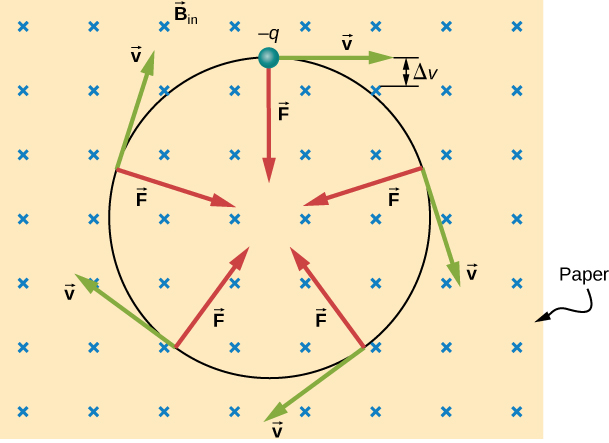In this situation, the magnetic force supplies the centripetal force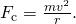Noting that the velocity is perpendicular to the magnetic field, the magnitude of the magnetic force is reduced to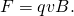Because the magnetic force F supplies the centripetal force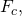we have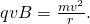Solving for r yields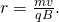Here, r is the radius of curvature of the path of a charged particle with mass m and charge q, moving at a speed v that is perpendicular to a magnetic field of strength B. The time for the charged particle to go around the circular path is defined as the period, which is the same as the distance traveled (the circumference) divided by the speed. Based on this and (Figure), we can derive the period of motion as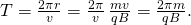If the velocity is not perpendicular to the magnetic field, then we can compare each component of the velocity separately with the magnetic field. The component of the velocity perpendicular to the magnetic field produces a magnetic force perpendicular to both this velocity and the field: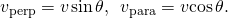where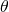is the angle between v and B. The component parallel to the magnetic field creates constant motion along the same direction as the magnetic field, also shown in (Figure). The parallel motion determines the pitch p of the helix, which is the distance between adjacent turns. This distance equals the parallel component of the velocity times the period: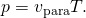The result is a helical motion, as shown in the following figure.

A charged particle moving with a velocity not in the same direction as the magnetic field. The velocity component perpendicular to the magnetic field creates circular motion, whereas the component of the velocity parallel to the field moves the particle along a straight line. The pitch is the horizontal distance between two consecutive circles. The resulting motion is helical.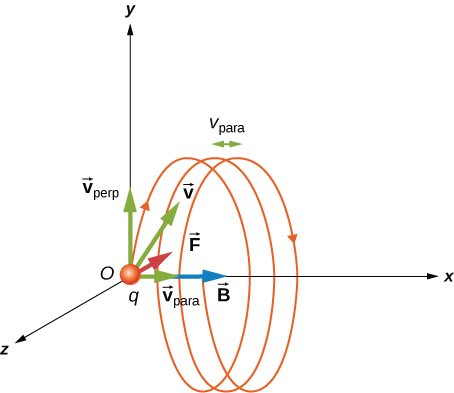While the charged particle travels in a helical path, it may enter a region where the magnetic field is not uniform. In particular, suppose a particle travels from a region of strong magnetic field to a region of weaker field, then back to a region of stronger field. The particle may reflect back before entering the stronger magnetic field region. This is similar to a wave on a string traveling from a very light, thin string to a hard wall and reflecting backward. If the reflection happens at both ends, the particle is trapped in a so-called magnetic bottle.

Trapped particles in magnetic fields are found in the Van Allen radiation belts around Earth, which are part of Earth’s magnetic field. These belts were discovered by James Van Allen while trying to measure the flux of cosmic rays on Earth (high-energy particles that come from outside the solar system) to see whether this was similar to the flux measured on Earth. Van Allen found that due to the contribution of particles trapped in Earth’s magnetic field, the flux was much higher on Earth than in outer space. Aurorae, like the famous aurora borealis (northern lights) in the Northern Hemisphere ((Figure)), are beautiful displays of light emitted as ions recombine with electrons entering the atmosphere as they spiral along magnetic field lines. (The ions are primarily oxygen and nitrogen atoms that are initially ionized by collisions with energetic particles in Earth’s atmosphere.) Aurorae have also been observed on other planets, such as Jupiter and Saturn.

(a) The Van Allen radiation belts around Earth trap ions produced by cosmic rays striking Earth’s atmosphere. (b) The magnificent spectacle of the aurora borealis, or northern lights, glows in the northern sky above Bear Lake near Eielson Air Force Base, Alaska. Shaped by Earth’s magnetic field, this light is produced by glowing molecules and ions of oxygen and nitrogen. (credit b: modification of work by USAF Senior Airman Joshua Strang)Beam Deflector A research group is investigating short-lived radioactive isotopes. They need to design a way to transport alpha-particles (helium nuclei) from where they are made to a place where they will collide with another material to form an isotope. The beam of alpha-particles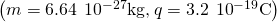bends through a 90-degree region with a uniform magnetic field of 0.050 T ((Figure)). (a) In what direction should the magnetic field be applied? (b) How much time does it take the alpha-particles to traverse the uniform magnetic field region?

Top view of the beam deflector setup.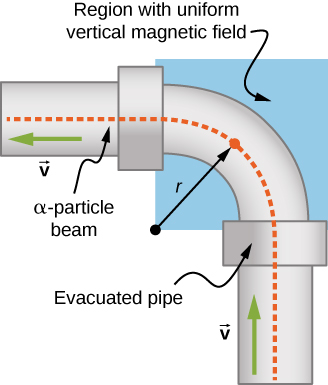Strategy

1. The direction of the magnetic field is shown by the RHR-1. Your fingers point in the direction of v, and your thumb needs to point in the direction of the force, to the left. Therefore, since the alpha-particles are positively charged, the magnetic field must point down.
2. The period of the alpha-particle going around the circle is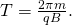Because the particle is only going around a quarter of a circle, we can take 0.25 times the period to find the time it takes to go around this path.

Solution

1. Let’s start by focusing on the alpha-particle entering the field near the bottom of the picture. First, point your thumb up the page. In order for your palm to open to the left where the centripetal force (and hence the magnetic force) points, your fingers need to change orientation until they point into the page. This is the direction of the applied magnetic field.
2. The period of the charged particle going around a circle is calculated by using the given mass, charge, and magnetic field in the problem. This works out to be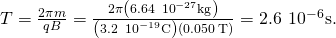However, for the given problem, the alpha-particle goes around a quarter of the circle, so the time it takes would be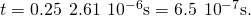Significance This time may be quick enough to get to the material we would like to bombard, depending on how short-lived the radioactive isotope is and continues to emit alpha-particles. If we could increase the magnetic field applied in the region, this would shorten the time even more. The path the particles need to take could be shortened, but this may not be economical given the experimental setup.

Check Your Understanding A uniform magnetic field of magnitude 1.5 T is directed horizontally from west to east. (a) What is the magnetic force on a proton at the instant when it is moving vertically downward in the field with a speed of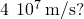(b) Compare this force with the weight w of a proton.

a.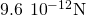toward the south; b.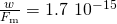Helical Motion in a Magnetic Field A proton enters a uniform magnetic field of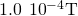with a speed of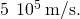At what angle must the magnetic field be from the velocity so that the pitch of the resulting helical motion is equal to the radius of the helix?

Strategy The pitch of the motion relates to the parallel velocity times the period of the circular motion, whereas the radius relates to the perpendicular velocity component. After setting the radius and the pitch equal to each other, solve for the angle between the magnetic field and velocity or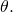Solution The pitch is given by (Figure), the period is given by (Figure), and the radius of circular motion is given by (Figure). Note that the velocity in the radius equation is related to only the perpendicular velocity, which is where the circular motion occurs. Therefore, we substitute the sine component of the overall velocity into the radius equation to equate the pitch and radius: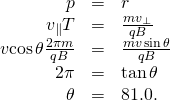Significance If this angle were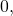only parallel velocity would occur and the helix would not form, because there would be no circular motion in the perpendicular plane. If this angle were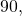only circular motion would occur and there would be no movement of the circles perpendicular to the motion. That is what creates the helical motion.

### Summary

• A magnetic force can supply centripetal force and cause a charged particle to move in a circular path of radius• The period of circular motion for a charged particle moving in a magnetic field perpendicular to the plane of motion is• Helical motion results if the velocity of the charged particle has a component parallel to the magnetic field as well as a component perpendicular to the magnetic field.

### Conceptual Questions

At a given instant, an electron and a proton are moving with the same velocity in a constant magnetic field. Compare the magnetic forces on these particles. Compare their accelerations.

The magnitude of the proton and electron magnetic forces are the same since they have the same amount of charge. The direction of these forces however are opposite of each other. The accelerations are opposite in direction and the electron has a larger acceleration than the proton due to its smaller mass.

Does increasing the magnitude of a uniform magnetic field through which a charge is traveling necessarily mean increasing the magnetic force on the charge? Does changing the direction of the field necessarily mean a change in the force on the charge?

An electron passes through a magnetic field without being deflected. What do you conclude about the magnetic field?

The magnetic field must point parallel or anti-parallel to the velocity.

If a charged particle moves in a straight line, can you conclude that there is no magnetic field present?

How could you determine which pole of an electromagnet is north and which pole is south?

A compass points toward the north pole of an electromagnet.

### Problems

A cosmic-ray electron moves at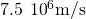perpendicular to Earth’s magnetic field at an altitude where the field strength is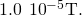What is the radius of the circular path the electron follows?

4.27 m

(a) Viewers of Star Trek have heard of an antimatter drive on the Starship Enterprise. One possibility for such a futuristic energy source is to store antimatter charged particles in a vacuum chamber, circulating in a magnetic field, and then extract them as needed. Antimatter annihilates normal matter, producing pure energy. What strength magnetic field is needed to hold antiprotons, moving at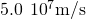in a circular path 2.00 m in radius? Antiprotons have the same mass as protons but the opposite (negative) charge. (b) Is this field strength obtainable with today’s technology or is it a futuristic possibility?

(a) An oxygen-16 ion with a mass of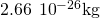travels at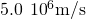perpendicular to a 1.20-T magnetic field, which makes it move in a circular arc with a 0.231-m radius. What positive charge is on the ion? (b) What is the ratio of this charge to the charge of an electron? (c) Discuss why the ratio found in (b) should be an integer.

a.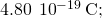b. 3; c. This ratio must be an integer because charges must be integer numbers of the basic charge of an electron. There are no free charges with values less than this basic charge, and all charges are integer multiples of this basic charge.

An electron in a TV CRT moves with a speed of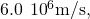in a direction perpendicular to Earth’s field, which has a strength of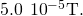(a) What strength electric field must be applied perpendicular to the Earth’s field to make the electron moves in a straight line? (b) If this is done between plates separated by 1.00 cm, what is the voltage applied? (Note that TVs are usually surrounded by a ferromagnetic material to shield against external magnetic fields and avoid the need for such a correction.)

(a) At what speed will a proton move in a circular path of the same radius as the electron in the previous exercise? (b) What would the radius of the path be if the proton had the same speed as the electron? (c) What would the radius be if the proton had the same kinetic energy as the electron? (d) The same momentum?

(a) 3.27 x 104 m/s (b) 12,525 m (c) 292 m (d) 6.83 m.

(a) What voltage will accelerate electrons to a speed of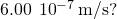(b) Find the radius of curvature of the path of a proton accelerated through this potential in a 0.500-T field and compare this with the radius of curvature of an electron accelerated through the same potential.

An alpha-particle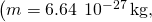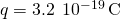travels in a circular path of radius 25 cm in a uniform magnetic field of magnitude 1.5 T. (a) What is the speed of the particle? (b) What is the kinetic energy in electron-volts? (c) Through what potential difference must the particle be accelerated in order to give it this kinetic energy?

a.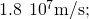b.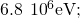c.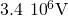A particle of charge q and mass m is accelerated from rest through a potential difference V, after which it encounters a uniform magnetic field B. If the particle moves in a plane perpendicular to B, what is the radius of its circular orbit?

### Glossary

cosmic rays
comprised of particles that originate mainly from outside the solar system and reach Earth
helical motion
superposition of circular motion with a straight-line motion that is followed by a charged particle moving in a region of magnetic field at an angle to the field